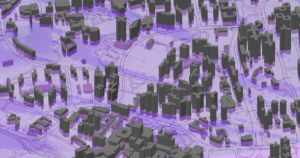# A Simplified Description of Coverage Probability Calculations for OFDM Systems in Radio Network Plan

Orthogonal Frequency Division Multiplexing (OFDM) is a technique that is used in many popular wireless communication systems, such as digital terrestrial broadcast systems (DVB-T, DVB-T2, ISDB-T, DTMB, CMMB, T-DAB, T-DMB), wireless LAN, WiMAX, and LTE.

OFDM works very well in radio channels where multipath propagation is particularly noticeable. Multipath propagation generates mainly two effects: intersymbol interference and frequency selective fading. By ensuring that the symbol duration is long enough, and using a guard interval between successive OFDM symbols, the system can handle the intersymbol interference caused by multipath propagation. Since wideband OFDM systems use multiple carrier frequencies, the frequency selective fading degrades only a few of the carriers. The information can then be retrieved by using interleaving and error correcting codes.

The ability of OFDM to overcome interference from multipath propagation enables transmission with multiple transmitters using the same frequency – Single Frequency Networks (SFN).

In a SFN multiple copies of the transmitted signals arrive at the receiving antenna with different amplitudes and delays. Strong diversity gains are then obtained resulting in better coverage and higher spectrum efficiency.In the receiver the signals are handled differently depending on their relative delay to the FFT synchronisation window. If the relative delay is shorter than the guard interval the signal is considered to be useful. If the relative delay is far outside the guard interval intersymbol interference will occur and the signal is considered to be interfering. If the relative delay is somewhere between the two extremes above the signal is considered to be both useful and interfering.In addition to the signals originated from the SFN transmitters, interfering signals from other transmitters will also be present at the receiver.

In cellular radio and broadcasting systems, where frequency channels are reused, the carrier-to-noise-and-interference ratio (CNIR) must be determined to be able to calculate the coverage probability. CNIR is the quotient between the sum of useful signal powers and the sum of the receiver noise and interfering signal powers.

In radio network planning software it is not feasibly to calculate the received signals in each location within the area to be considered. The signal strength from each transmitter is estimated by a radio propagation model on a regular grid of points. Usually, this grid will have the same resolution as the terrain databases used by the radio propagation model. As a consequence, each grid point can be seen as an area of corresponding size, for example 50×50 meter. These small areas are called pixels. Within each pixel the received useful and interfering signals are assumed to be random variables with lognormal distributions. The CNIR ratio is then determined each pixel by doing a summation of random variables. The coverage probability in each pixel is determined by calculating the probability that the CNIR distribution exceeds a system specific planning value.

At right you see a coverage probability map in 3D produced by using PROGIRA® plan. Thanks to my colleague Björn Tannersjö for the production of this map.

If you have any questions about Radio Network Planning, OFDM, SFN, Coverage Probability Calculations, or if you have any other questions, don’t hesitate to contact me.